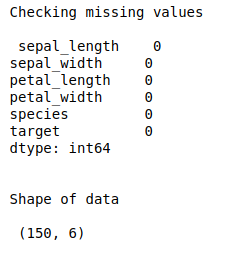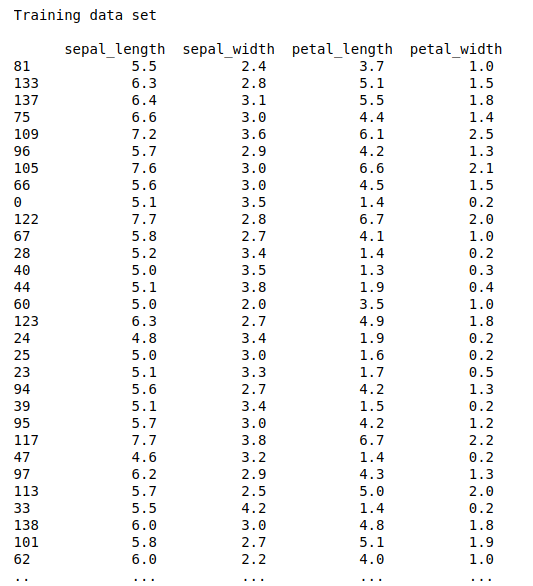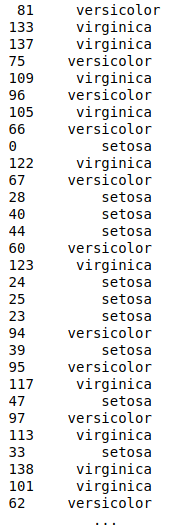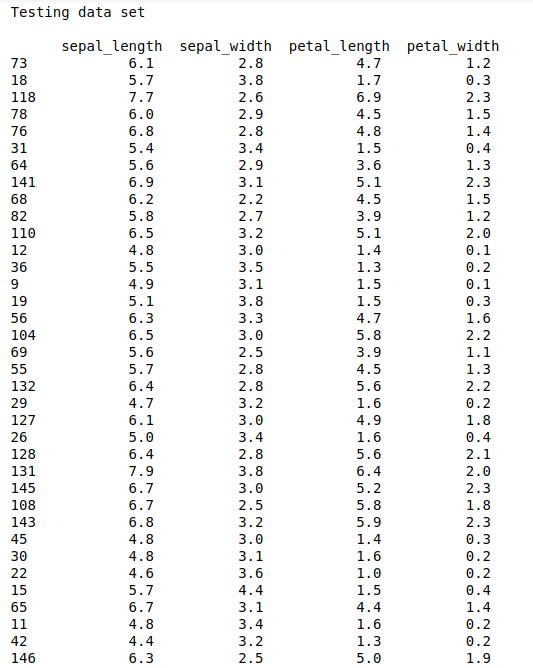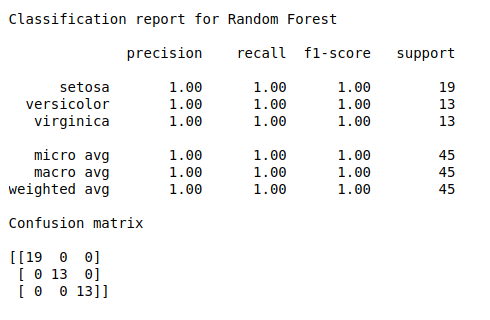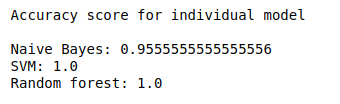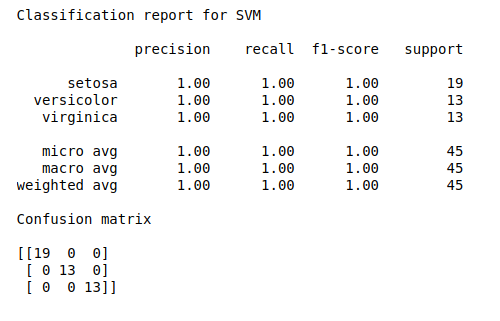Confusion matrix.

Accuracy.

Classification report.

"/>

• #5, First Floor, 4th Street Dr. Subbarayan Nagar Kodambakkam, Chennai-600 024 Landmark : Samiyar Madam
• pro@slogix.in
• +91- 81240 01111

#### Social List

##### How to build a ensemble of machine learning classifiers in python?
###### Description

To build a ensemble model for improved results using Voting classifier technique in python

###### Process

Set the dependent and independent features.

Split the data into training and testing set.

Build the base estimators.

Import Voting Classifier from sklearn library.

Fit the data into the model.

Predict the test data.

Calculate accuracy, precision and recall

###### Sapmle Code

#import libraries
import warnings
warnings.filterwarnings(“ignore”)
import pandas as pd
import numpy as np
from sklearn.model_selection import train_test_split
from sklearn import model_selection
from sklearn.model_selection import cross_val_score
from sklearn.naive_bayes import MultinomialNB
from sklearn.svm import SVC
from sklearn.ensemble import RandomForestClassifier
from sklearn.model_selection import GridSearchCV
from sklearn.ensemble import VotingClassifier
from sklearn.metrics import classification_report, confusion_matrix, accuracy_score

#check missing values
print(“Checking missing values\n\n”,data.isnull().sum())

#make it as a data frame
df = pd.DataFrame(data)

#print data shape
print(“\n”)
print(“Shape of data\n\n”,df.shape)

#Feature extraction
#Define X and y variable
X = df.iloc[:,0:4]
y = df.iloc[:,4]

#Training and testing data using 80:20 rule
#Split train and test data
X_train, X_test, y_train, y_test = train_test_split(X, y, test_size=0.3, random_state=42)

#training set and testing set
print(“\n”)
print(“Training data set\n\n”,X_train,”\n”,y_train)
print(“\n”)
print(“Testing data set\n\n”,X_test)

#Building the model

#Naive-bayes
naive_bayes = MultinomialNB()
naive_bayes.fit(X_train,y_train)
y_pred1 = naive_bayes.predict(X_test)

#Evaluate the model
print(“\n”)
print(“Classification report for Naive_bayes\n”)
print(classification_report(y_test, y_pred1))
print(“Confusion matrix\n”)
print(confusion_matrix(y_test, y_pred1))
print(“\n”)
#print(“Accuracy score”)
#print(accuracy_score(y_test, y_pred1))
#print(“\n”)

#SVM
svm = SVC(kernel=’linear’,probability=True)
svm.fit(X_train,y_train)
y_pred2 = svm.predict(X_test)

#Evaluate the model
print(“\n”)
print(“Classification report for SVM\n”)
print(classification_report(y_test, y_pred2))
print(“Confusion matrix\n”)
print(confusion_matrix(y_test, y_pred2))
print(“\n”)
#print(“Accuracy score”)
#print(accuracy_score(y_test, y_pred2))
#print(“\n”)

#Random forest
rf = RandomForestClassifier()
#create a dictionary of all values we want to test for n_estimators
params_rf = {‘n_estimators’: [50, 100, 200]}
#use gridsearch to test all values for n_estimators
rf_gs = GridSearchCV(rf, params_rf, cv=5)
#fit model to training data
rf_gs.fit(X_train, y_train)
rf_best = rf_gs.best_estimator_
y_pred5 = rf_best.predict(X_test)

#Evaluate the model
print(“\n”)
print(“Classification report for Random Forest\n”)
print(classification_report(y_test, y_pred5))
print(“Confusion matrix\n”)
print(confusion_matrix(y_test, y_pred5))
print(“\n”)
#print(“Accuracy score”)
#print(accuracy_score(y_test, y_pred5))
#print(“\n”

#Score for individual model
print(“Accuracy score for individual model\n”)
print(‘Naive Bayes: {}’.format(naive_bayes.score(X_test, y_test)))
print(‘SVM: {}’.format(svm.score(X_test, y_test)))
print(‘Random forest: {}’.format(rf_best.score(X_test, y_test)))

#create a dictionary of our models
estimators=[(‘Bayes’,naive_bayes),(‘svm’,svm),(‘rf’, rf_best)]

#create our voting classifier, inputting our models
ensemble = VotingClassifier(estimators, voting=’soft’)

#fit model to training data
ensemble.fit(X_train, y_train)
y_pred6 = ensemble.predict(X_test)

#Evaluate the model
print(“\n”)
print(“Classification report for Ensemble model\n”)
print(classification_report(y_test, y_pred6))
print(“Confusion matrix\n”)
print(confusion_matrix(y_test, y_pred6))
print(“\n”)

#test our model on the test data
print(“Accuracy score of ensemble model “)
print(‘Ensemble: {}’.format(ensemble.score(X_test, y_test)))

###### Screenshots